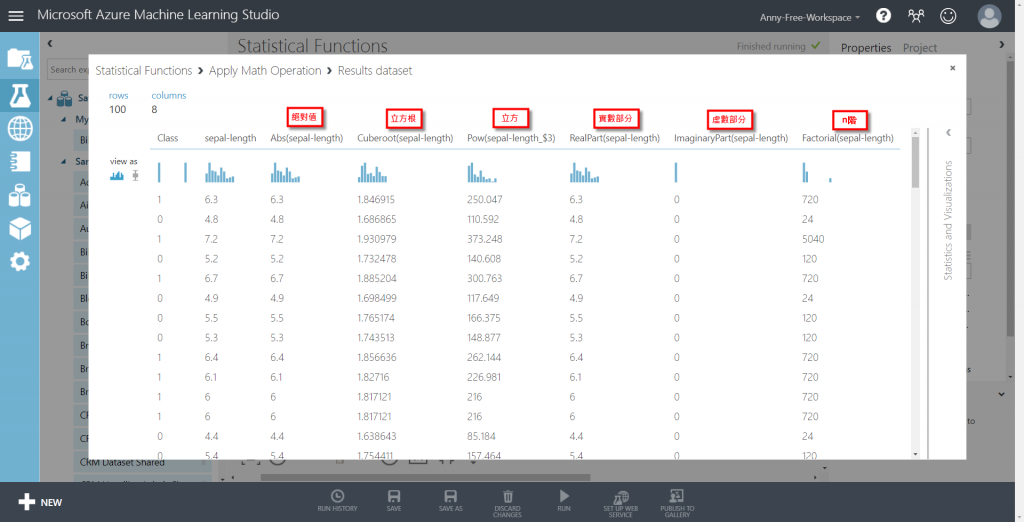#2019 iT 邦幫忙鐵人賽DAY 6
0
Everything on Azure

## Azure Machine Learning Studio 資料前處理 - 數學運算 Apply Math Operation (1) 基礎 Basic

Statistical Functions 統計函數有以下 7 種：

1. Apply Math Operation 數學運算
2. Compute Elementary Statistics 計算基礎統計
3. Compute Linear Correlation 計算線性相關
4. Evaluate Probability Function 評估機率函數
5. Replace Discrete Values 替換離散值
6. Summarize Data 彙總資料
7. Test Hypothesis using t-Test 統計假設檢定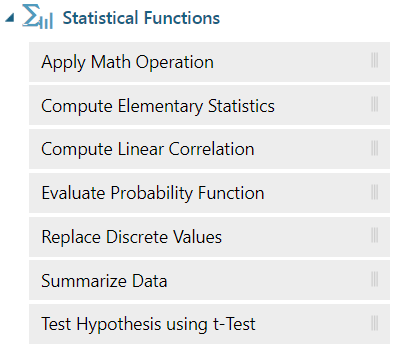## Apply Math Operation 數學運算

Apply Math Operation 數學運算包含以下 6 種：

1. Basic 基礎
2. Compare 比較
3. Operations 運算
4. Rounding 四捨五入
5. Special 特殊
6. Trigonometric 三角函數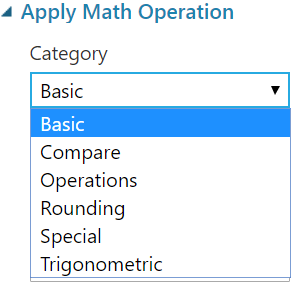1. Append 加入為新欄位
2. Inplace 取代原有欄位
3. ResultOnly 只留下運算結果欄位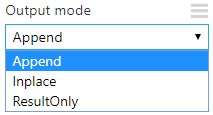### Basic 基礎運算

• Abs 絕對值
• Atan2 反正切三角函數
• Conj 共軛函數
• CubeRoot 立方根
• DpubleFactorial 雙階乘(n!!)
• Eps 最小浮點數精度
• Exp 以e為基底的指數
• Exp2 以2為基底的指數
• ExpMinus1 負指數
• Factorial 階乘(n!)
• Hypotenuse 三角函數斜邊計算
• ImaginaryPart 複數的虛數部分
• Ln 一個數的自然對數
• LnPlus1 Ln(X+1)
• Log 對數函數
• Log10 以10為底的對數函數
• Log2 以2為底的對數函數
• NthRoot 開根號
• Pow 次方
• RealPart 複數的實數部分
• Sqrt 平方根
• SqrtPi 乘 π 開根號
• Square 平方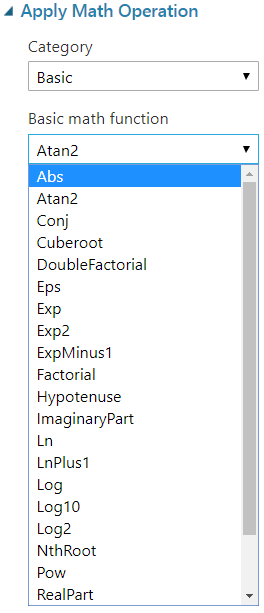## Apply Math Operation - Basic 基礎運算

(1) 以鳶尾花分類資料集為例，新增"Apply Math Operation"數學運算，將資料集輸出接至 Apply Math Operation 的輸入，選擇 Basic 基礎運算，選擇運算函式 cuberoot 開立方根，接著選擇要做運算的欄位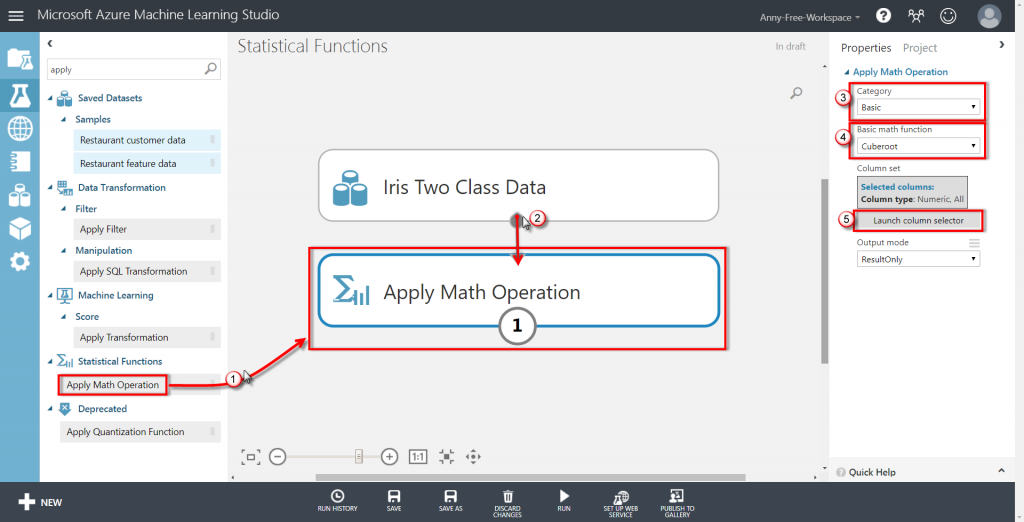(2) 這裡選擇 sepal-length 花萼長度，點選右下角勾勾確認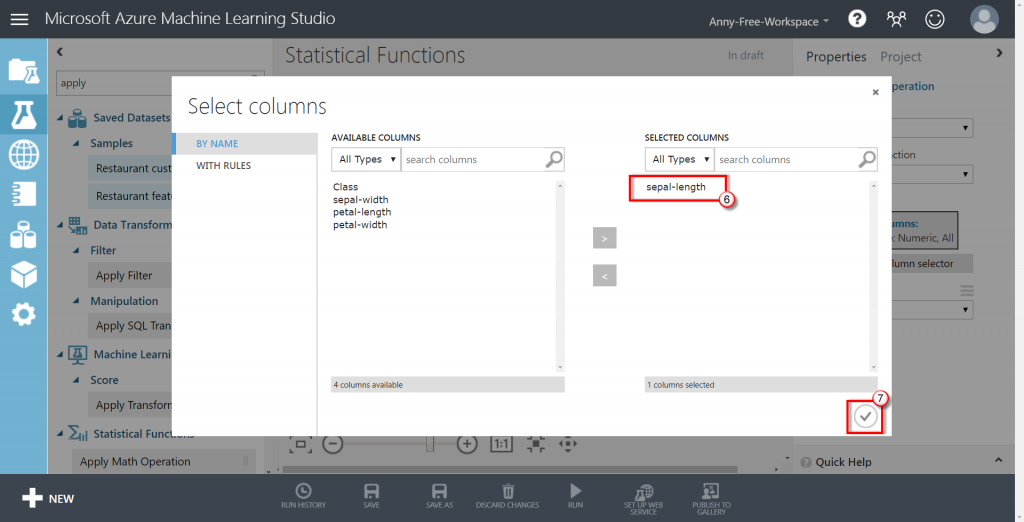(3) 選擇運算結果處理方式 Append 加入新欄位，點選 Run 執行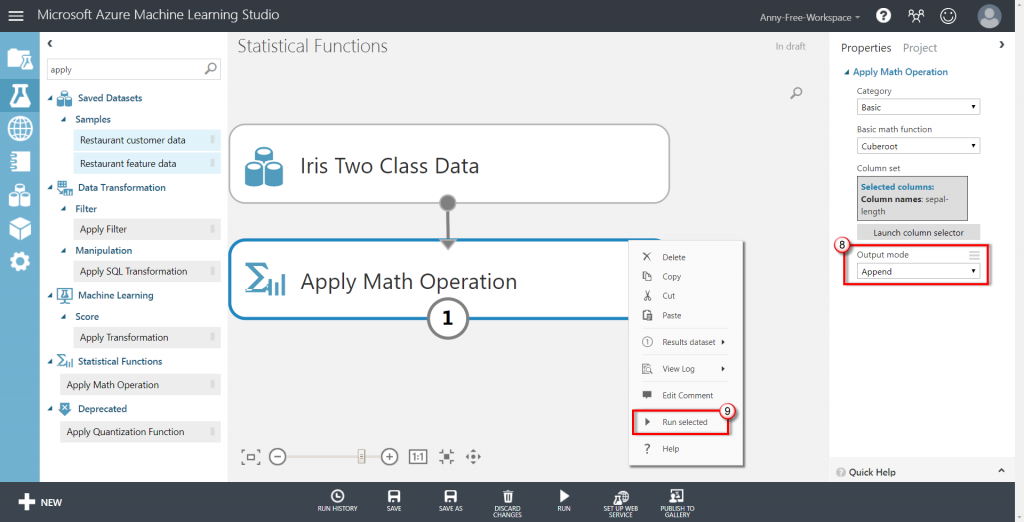(4) 執行完成，可以點選滑鼠右鍵檢視結果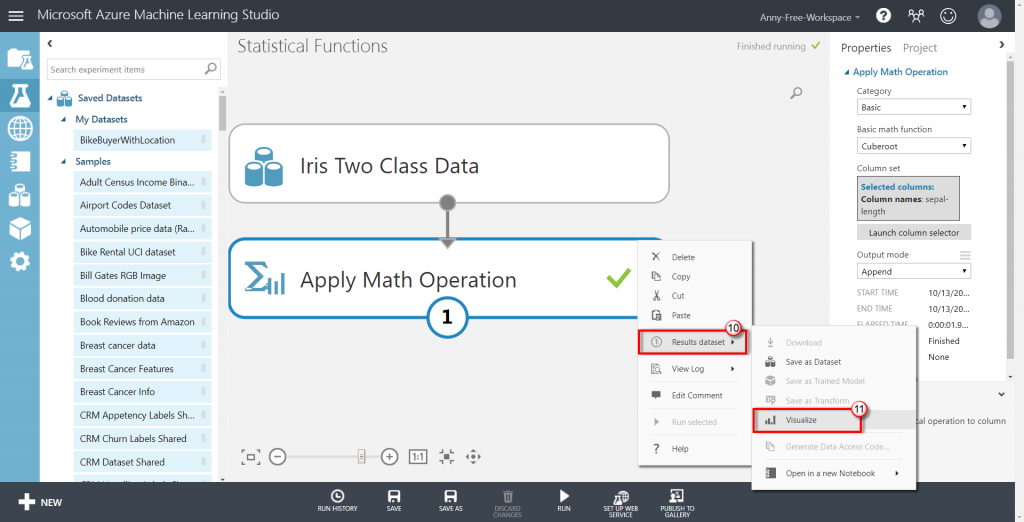(5) 可以看到運算結果被加在最後一個欄位 Cubroot(sepal-length)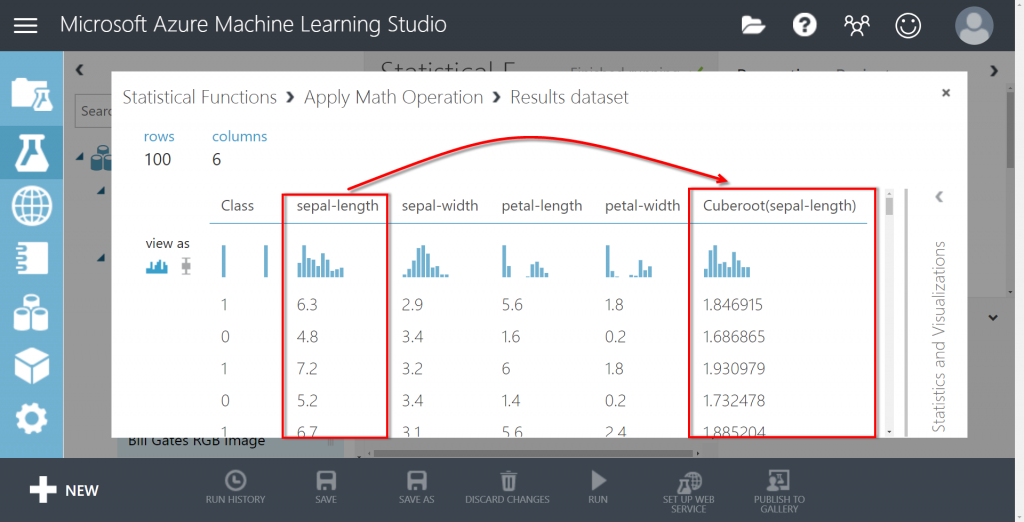(6) 其他的函數也是類似作法，這邊針對相同欄位 sepal-length 花萼長度試了幾個不同的基礎運算的結果如下